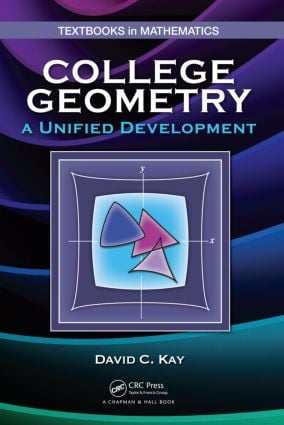# College Geometry

## A Unified Development, 1st Edition

CRC Press

652 pages | 657 B/W Illus.

Hardback: 9781439819111
pub: 2011-06-24
SAVE ~\$23.00
\$115.00
\$92.00
x
eBook (VitalSource) : 9780429109195
pub: 2011-06-24
from \$57.50

FREE Standard Shipping!

### Description

Designed for mathematics majors and other students who intend to teach mathematics at the secondary school level, College Geometry: A Unified Development unifies the three classical geometries within an axiomatic framework. The author develops the axioms to include Euclidean, elliptic, and hyperbolic geometry, showing how geometry has real and far-reaching implications. He approaches every topic as a fresh, new concept and carefully defines and explains geometric principles.

The book begins with elementary ideas about points, lines, and distance, gradually introducing more advanced concepts such as congruent triangles and geometric inequalities. At the core of the text, the author simultaneously develops the classical formulas for spherical and hyperbolic geometry within the axiomatic framework. He explains how the trigonometry of the right triangle, including the Pythagorean theorem, is developed for classical non-Euclidean geometries. Previously accessible only to advanced or graduate students, this material is presented at an elementary level. The book also explores other important concepts of modern geometry, including affine transformations and circular inversion.

Through clear explanations and numerous examples and problems, this text shows step-by-step how fundamental geometric ideas are connected to advanced geometry. It represents the first step toward future study of Riemannian geometry, Einstein’s relativity, and theories of cosmology.

### Reviews

"The book is a comprehensive textbook on basic geometry. … Key features of the book include numerous figures and many problems, more than half of which come with hints or even complete solutions. Frequent historical comments add to making the reading a pleasant one."

—Michael Joswig, Zentralblatt MATH 1273

Lines, Distance, Segments, and Rays

Intended Goals

Axioms of Alignment

A Glimpse at Finite Geometry

Metric Geometry

Eves’ 25-Point Affine Geometry: A Model for Axioms 0–4

Distance and Alignment

Properties of Betweenness: Segments and Rays

Coordinates for Rays

Geometry and the Continuum

Segment Construction Theorems

Angles, Angle Measure, and Plane Separation

Angles and Angle Measure

Plane Separation

Consequences of Plane Separation: The Postulate of Pasch

The Interior of an Angle: The Angle Addition Postulate

Angle Construction Theorems

Consequences of a Finite Metric

Unified Geometry: Triangles and Congruence

Congruent Triangles: SAS Hypothesis

A Metric for City Centers

The SAS Postulate and the ASA and SSS Theorems

Euclid’s Superposition Proof: An Alternative to Axiom 12

Locus, Perpendicular Bisectors, and Symmetry

The Exterior Angle Inequality

Inequalities for Triangles

Further Congruence Criteria

Special Segments Associated with Triangles

The Quadrilaterals of Saccheri and Lambert

Polygons

Circles in Unified Geometry

Three Geometries

Parallelism in Unified Geometry and the Influence of α

Elliptic Geometry: Angle-Sum Theorem

Pole-Polar Theory for Elliptic Geometry

Angle Measure and Distance Related: Archimedes’ Method

Hyperbolic Geometry: Angle-Sum Theorem

A Concept for Area: AAA Congruence

Parallelism in Hyperbolic Geometry

Asymptotic Triangles in Hyperbolic Geometry

Euclidean Geometry: Angle-Sum Theorem

Median of a Trapezoid in Euclidean Geometry

Similar Triangles in Euclidean Geometry

Pythagorean Theorem

An Inequality Concept for Unified Geometry

Ratio Inequalities for Trapezoids

Ratio Inequalities for Right Triangles

Orthogonal Projection and "Similar" Triangles in Unified Geometry

Unified Trigonometry: The Functions c(θ) and s(θ)

Trigonometric Identities

Classical Forms for c(θ) and s(θ)

Lambert Quadrilaterals and the Function C(u)

Identities for C(u)

Classical Forms for C(u)

The Pythagorean Relation for Unified Geometry

Classical Unified Trigonometry

Beyond Euclid: Modern Geometry

Directed Distance: Stewart’s Theorem and the Cevian Formula

Formulas for Special Cevians

Circles: Power Theorems and Inscribed Angles

Using Circles in Geometry

Cross Ratio and Harmonic Conjugates

The Theorems of Ceva and Menelaus

Families of Mutually Orthogonal Circles

Transformations in Modern Geometry

Projective Transformations

Affine Transformations

Similitudes and Isometries

Line Reflections: Building Blocks for Isometries and Similitudes

Translations and Rotations

Circular Inversion

Non-Euclidean Geometry: Analytical Approach

Law of Sines and Cosines for Unified Geometry

Unifying Identities for Unified Trigonometry

Half-Angle Identities for Unified Geometry

The Shape of a Triangle in Unified Geometry: Cosine Inequality

The Formulas of Gauss: Area of a Triangle

Directed Distance: Theorems of Menelaus and Ceva

Poincarè’s Model for Hyperbolic Geometry

Other Models: Surface Theory

Hyperbolic Parallelism and Bolyai’s Ideal Points

Appendix B: Intuitive Spherical Geometry

Appendix C: Proof in Geometry

Appendix D: The Real Numbers and Least Upper Bound

Appendix F: Axiom Systems for Geometry

Solutions to Selected Problems

Bibliography

Index

Now retired, David C. Kay was a professor and chairman of the Department of Mathematics at the University of North Carolina–Asheville for 14 years. He previously taught at the University of Oklahoma for 17 years. His research interests include distance geometry, convexity theory, and related functional analysis.